# Diffusion MRI: Curve Inference and Streamline Flows

### Introduction

In this work, we apply notions from Computer Vision and Perceptual Organisation to the problem of modeling biological tissue structure in diffusion MRI data. By measuring the local Brownian motion of water molecules, diffusion MRI provides estimates of local fibre orientations in tissues such as the white matter of the brain. For an introduction to diffusion MRI, please click here. Over the last years, diffusion MRI has become an important tool for the in vivo study of brain connectivity. Nevertheless, the inference of the structure of white matter fibres is still an open problem.

The key ideas in this work are to model white matter fibres as 3D space curves, to view diffusion MRI data as providing information about the tangent vectors of these curves, and to frame the problem as that of inferring 3D curve geometry from a discretized, incomplete, and potentially blurred and noisy field of tangent measurements. Inspired by notions used in Perceptual Organisation in Computer Vision, we develop local geometrical constraints which guide the inference process and ultimately result in the recovery of the underlying fibre geometry.

### Background

#### Perceptual Organisation

The human visual system has the remarkable ability to perceive structure even in what may appear at first sight as unordered visual stimuli, such as the following visual illusions, in which the circles (three in the left image, one in the right) are easily distinguishable despite the cluttered background.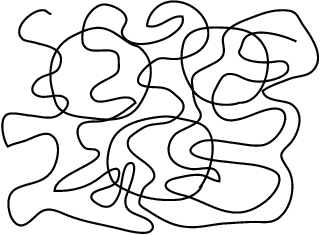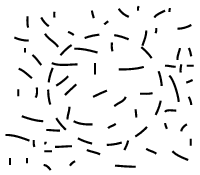This ability to impose a certain structural organisation on sensory data, so that sensory primitives that arise from common causes are grouped together, is refered to as "Perceptual Organisation". It has been widely studied in psychology and vision science since the beginning of the XXth century.

#### 2D Curve Inference

When a 3D space curve is imaged, it is projected onto the 2D image plane. But this planar projection of the curve is not directly observable in the image. Since images represent intensity values over a plane quantized into a set of pixels, the only information available is related to the trace of the curve, i.e., the set of pixels through which it passes. But even the trace is not directly available in images, since it needs to be first segmented. Because of this information loss inherent to the imaging process, the problems of recovering the projected curve is ill-posed. However, smoothness assumptions permit the inference of trace points, tangents and curvatures. In , Parent and Zucker argue that minimizing the variation of curvature along a curve is necessary and sufficient to design such an inference procedure, refered to as 2D curve inference.

The inference method  proceeds by first estimating a coarse description of the local (quantized) differential geometry of the curve, by convolving the image with a set of linear operators that provide a set of initial tangent estimates at each position. Then, since one of the goals is to infer local curvature, the osculating circle is adopted as a local approximation to the curve in a neighborhood around each point, as illustrated below:Here, the osculating circle (red) provides a model for the local transport of curve tangents (green). In this example, the two leftmost tangents are co-circular, i.e., they are mutually related via tangency to the same osculating circle.

2D curve inference is based upon the inference of the best osculating circle at each point, with the goal of minimizing curvature variation by maximizing circularity. This measure of circularity in a neighborhood is established in terms of a pairwise relationship between (estimated) tangent elements, which is refered to as co-circularity. Its definition is as follows : two unit tangents are co-circular if and only if there exists a circle to which both are tangent.

The next step is to determine a support measure between tangent estimates in a neighborhood. This measure is based on the co-circularity constraint, and is precisely defined in . It is then incorporated in a relaxation labeling algorithm , with the result that at each pixel, those tangents that are supported by other nearby orientations via the co-circularity model gain confidence, while others are suppressed. The end result is the emergence of the curve structures in the image. See  for more details.

#### 2D Texture Flow Inference

Ben-Shahar and Zucker  define a "texture flow" as a set of locally parallel curves with a slowly varying local orientation in the image plane. This type of pattern is common in both natural and man-made scenes. As it can be seen by the examples presented next, it often results from the imaging of 3D curve-like structures such as the threads in a yarn ball, the stripes on a zebra, or a wheat field.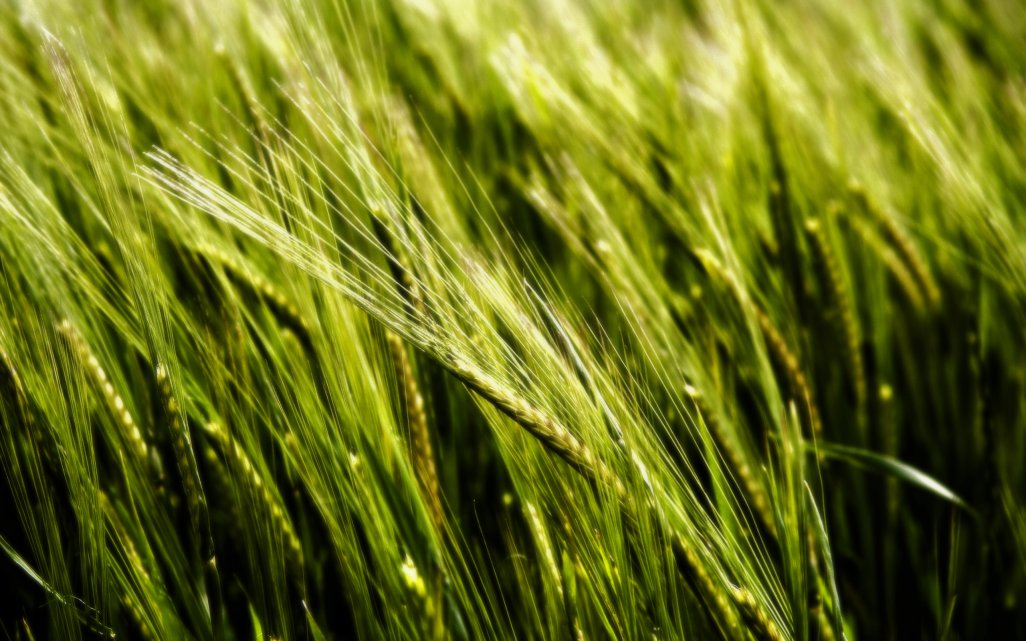As exemplified by the following detail of an engraving by Albrecht Dürer, such patterns may play an important role in our perception of 3D shape and shading, and have been judiciously used by artists to render shape.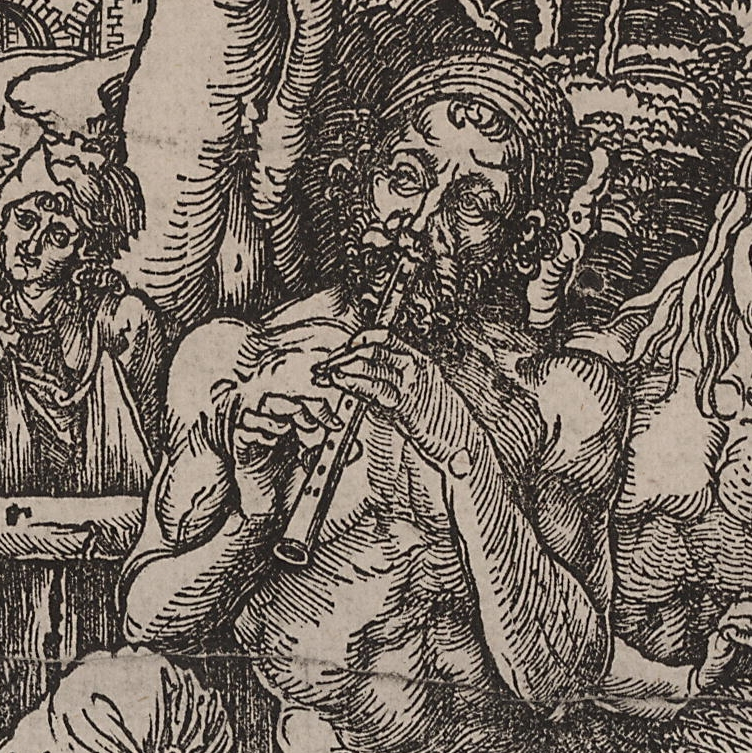Mathematically, a texture flow may be represented as an orthonormal frame field (ET ,EN), where ET and EN are mutually orthogonal unit vectors, defined at each point (x,y), such that ET is tangent to the flow, as illustrated here:In this representation, θ(x,y) is the angle between ET and the x axis. Given that texture flow patterns are continuous, and their characteristic property, orientation, is local, a differential analysis is applied in  in order to understand and define the parameters that govern the local behaviour of a texture flow. In particular, the differential behaviour of a (ET , EN) frame is examined as it moves within a small neighbourhood over the image plane. This leads to the definition of two curvature functions, the tangential and the normal curvature. In an object centered representation, the tangential curvature measures the rate of change of ET in the tangential direction, and the normal curvature measures the rate of change of ET in the normal direction EN.

In the 2D curve inference procedure, the goal was to infer likely curve trace from a set of curve tangent estimates distributed over a 2D image. This was achieved through a co-circularity constraint, imposed through the use of a circle as a local osculating object to the curve trace to be infered. In , the goal is to develop a procedure for the inference of texture flow patterns in a 2D image, once again based on an initial set of tangent estimates distributed over the image. If the circle is an intuitive choice for a local osculating object for a 2D curve, the choice of an osculating object for a texture flow pattern is less obvious. The idea in the 2D curve inference case was that nearby curve tangents should be related by a constant curvature measure. In the 2D texture flow case, unfortunately it is not possible to keep both the tangential and the normal curvatures constant simultaneously (except in the trivial case when both equal 0), as proven in . However, it is possible to require a constant co-variation of the two curvatures, by enforcing a constant ratio between them at the point where they are measured. This constant ratio constraint, together with energy minimization considerations, motivate the choice of the right helicoid as an osculating object. The helicoid is known to be the only ruled minimal surface in Euclidean 3D space other than the plane. For a description of the mathematical properties of the helicoid surface, please click here.

The right helicoid object provides a local model for a coherent texture flow, and it allows to assess the degree to which a particular flow tangent measurement is consistent with the context in which it is embedded. This can be used to infer âbest-fitâ local models for a general texture flow pattern, as well as in refining noisy measurements and removing spurious ones. Just as with the case of 2D curve inference, these tasks are achieved by embedding the texture flow model in a relaxation labeling framework. Based on the right-helicoid osculating object, a support function is defined, where compatibility is measured with respect to an underlying texture flow model, discretized in a range of values for the tangential and normal curvatures. See  for more details.

### 3D Curve Inference

In our work described in , we extend the 2D curve inference ideas to 3D and we then apply them to diffusion MRI data. The resulting algorithm is refered to as 3D curve inference. Based on the geometrical properties of the helix curve, a justification is made in  for the use of local helical approximations to the general curves that represent white matter fibres. A notion of co-helicity between triplets of orientation estimates at distinct locations is introduced and incorporated in a method for the inference of the best-fit local helical approximations of the white matter fibres. This allows for local curvature and torsion estimates to be obtained. The inferred geometry is then used to regularize diffusion MRI data, and an improvement in the performance of a fibre tracking algorithm is demonstrated. The methods are validated on data acquired in vivo from a human brain, on data from a biological phantom, and on synthetic data.

The notion of co-helicity between a set of three tangents specified at three locations allows us to obtain estimates of the trace, tangent, curvature and torsion fields of curves in the 3D volume. This is the natural extension to 3D of the above co-circularity constraint. In this framework, an osculating helix (which has constant curvature and constant torsion) is used to locally approximate a curve passing through a given location. A regularization algorithm is then implemented, such that tangents that are supported by other tangents in their neighbourhood via the co-helicity relation gain confidence, while others are suppressed. The following illustration presents a schematic of this process.The left panel of this figure shows a hypothetical arrangement of diffusion orientation distribution functions (ODFs). The right panel illustrates the effect of the 3D curve inference algorithm of 1) inferring curves (dashed lines) to model the underlying fibres, and, 2) reshaping the ODFs according to the inferred curves. ODF values in directions that are not aligned with the inferred curves are suppressed, whereas those that are best aligned with the inferred curves are maximally supported. Thus, the central ODF obtains an Χ shape to reflect the underlying crossing. The blue ODF maxima are tangent to the same helical curve and are thus co-helical. Similarly, the red ODF maxima are tangent to the other helical curve and are thus co-helical as well.

The following are examples of regularization results obtained on in vivo human brain data using 3D curve inference. For more examples and further details, please consult .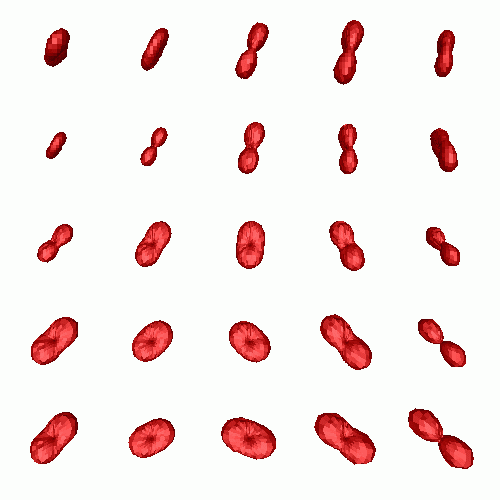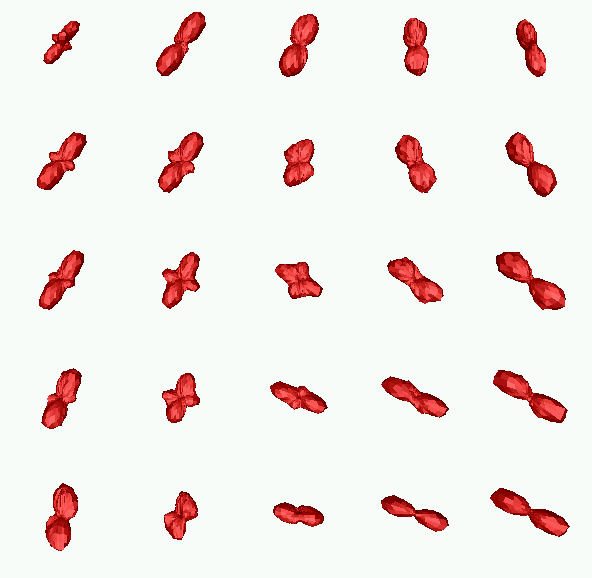The top row shows (from left to right): the original diffusion tensors (DT) (after subtraction of the maximally inscribed sphere for better visualization, see ), and the result obtained after applying 3D curve inference regularization. The bottom row shows (from left to right): the original Q-Ball (QB) ODF reconstruction, the result obtained after applying 3D curve inference regularization, and the location of the region of interest (ROI) where all these results are observed. We observe that the unregularized diffusion tensors are ambiguous in voxels where partial volume averaging of directions occurs. In contrast, both the QB and the regularized DT ODFs exhibit multiple maxima in these voxels that are qualitatively very similar to each other. Finally, one can see that 3D curve inference sharpens the QB ODFs and makes their maxima salient. In , we provide fibre tractography results, as well as validation results on a biological phantom and on synthetic data.

Here is an example of how regularization by 3D curve inference may impact fibre tractography.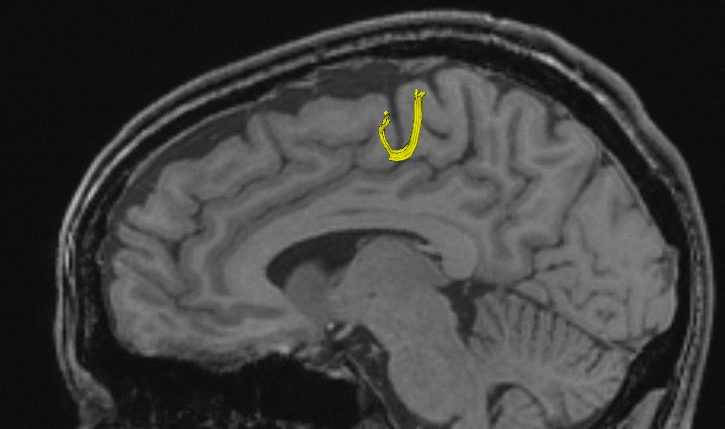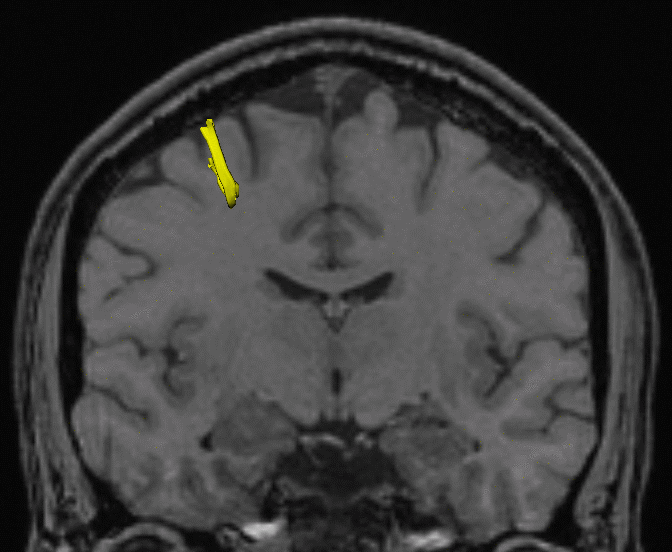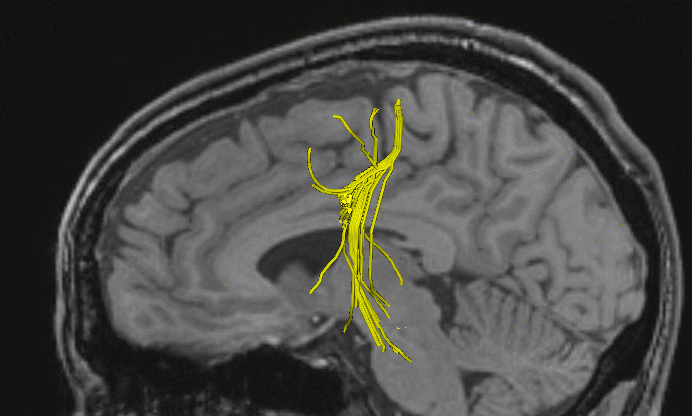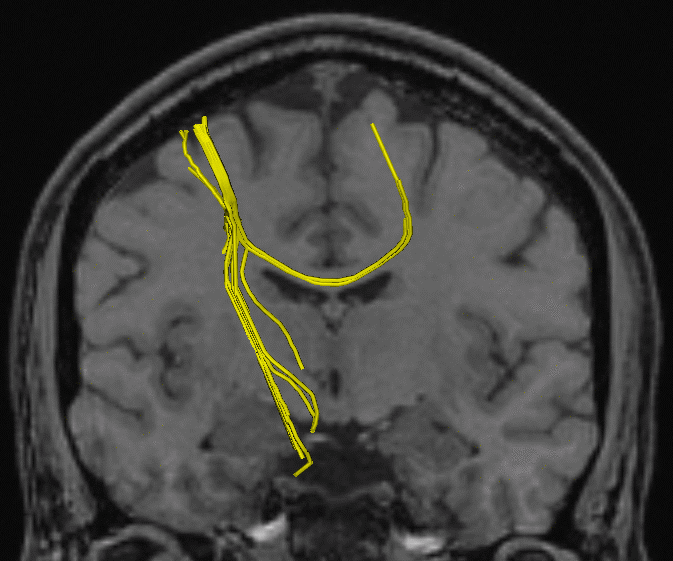To generate the above figures, a streamline fibre tracking algorithm was initialized in a small seed region near the cortex. In the top row, tracking was run on unregularized diffusion tensors. As soon as it reaches a regions of partial volume averaging effects, tracking stops. After regularization, the tracking algorithm can cross the region of complex fibre architecture (bottom row), and follow the callosal projections to the other hemisphere, as well as the cortico-spinal tract down to the spine. See  for more details.

### Characterization of fibre sub-voxel geometry

In recent work published in , we develop a method for the labeling of complex sub-voxel fibre configuration in high angular resolution diffusion MRI (HARDI). HARDI presents numerous advantages over the tensor model and can be used to achieve better precision in fibre tractography. However, the relationship between the measured diffusion probability density function (pdf) and tissue structure remains ambiguous. Furthermore, certain subvoxel fibre configurations can result in ODF shapes that are difficult to interpret during tractography. Different fibre geometries can yield similar ODFs, but require different actions to be taken in tractography. To illustrate this fact, consider the two types of sub-voxel fibre structures schematically depicted here: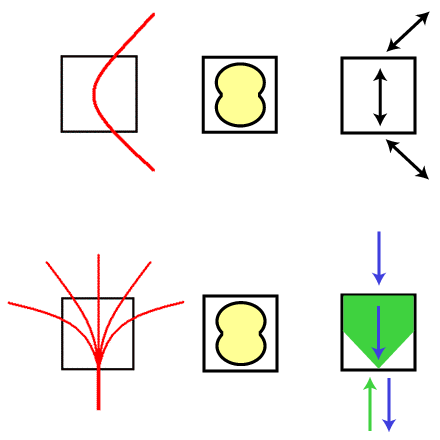In this example, both the single curving fibre tract (top row) and the fanning fibre tract (bottom row) are likely to result in an almost undistinguishable ODF with a single broad peak oriented in the vertical direction (middle panel in both rows). This is due to the presence of a relatively wide array of fibre tangent directions within one voxel, which results in a broad fibre ODF profile. Although they yield a similar ODF, each of these structures requires a different action to be taken by a fibre tracking algorithm. In the case of a curving fibre bundle, only one curve (or streamline) should be propagated, in the medial direction of the broad maximum. In the case of a fan configuration, multiple curves should be followed when propagating in one direction (represented by a polarity vector), but only one direction should be followed when propagating in the other. Hence, in order to take the appropriate action, it is crucial for tracking methods to be able to differentiate between these types of sub-voxel configurations.

In , we apply the 3D curve inference algorithm in a labeling scheme that disambiguates fibre ODFs that result from the following possible configurations:
1. Single fibre bundles - These may exhibit curvature and torsion at a sub-voxel scale.
2. Fanning fibre tracts - Where a single fibre bundle fans into multiple directions at a subvoxel scale.
3. Crossing fibre tracts - Where two or more (possibly curving) fibre tracts cross within a voxel.
This is achieved through an analysis of the local configurations of helical curve approximations, inferred through the 3D curve inference algorithm at each voxel. 3D curve inference integrates information over a neighborhood, which allows for the resolution of ambiguities that cannot be resolved by considering ODF shape alone.

Our labeling method based on 3D curve inference allows us to observe results such as the following: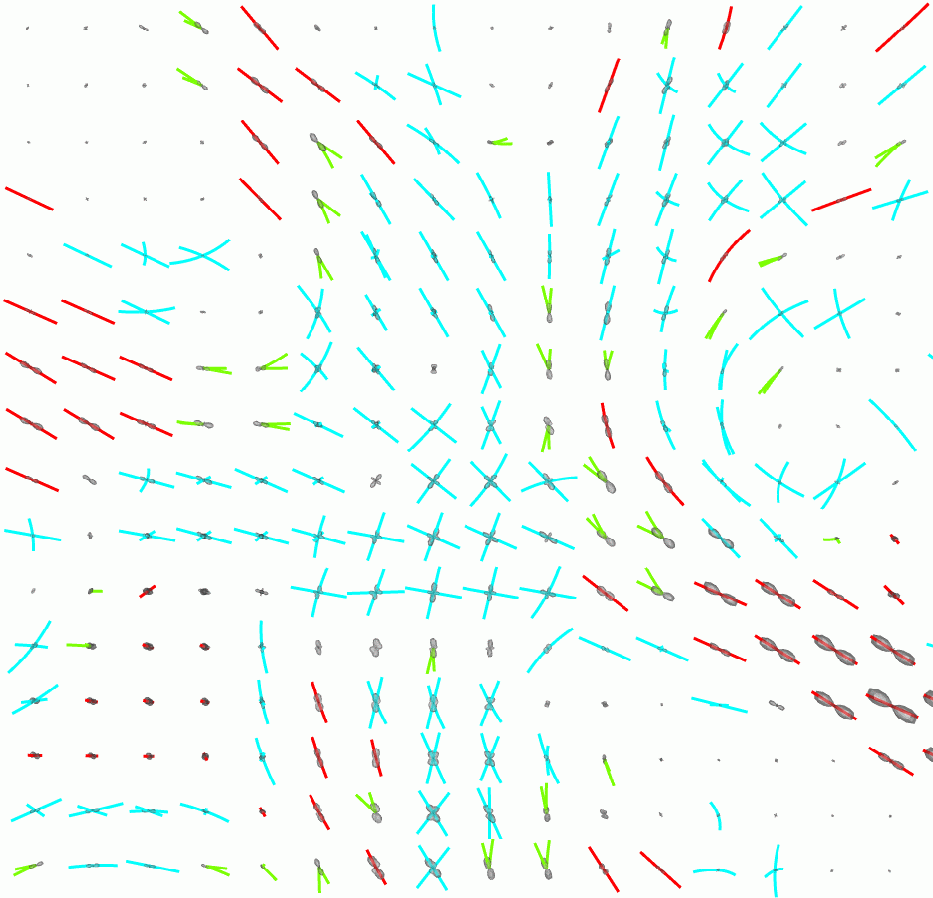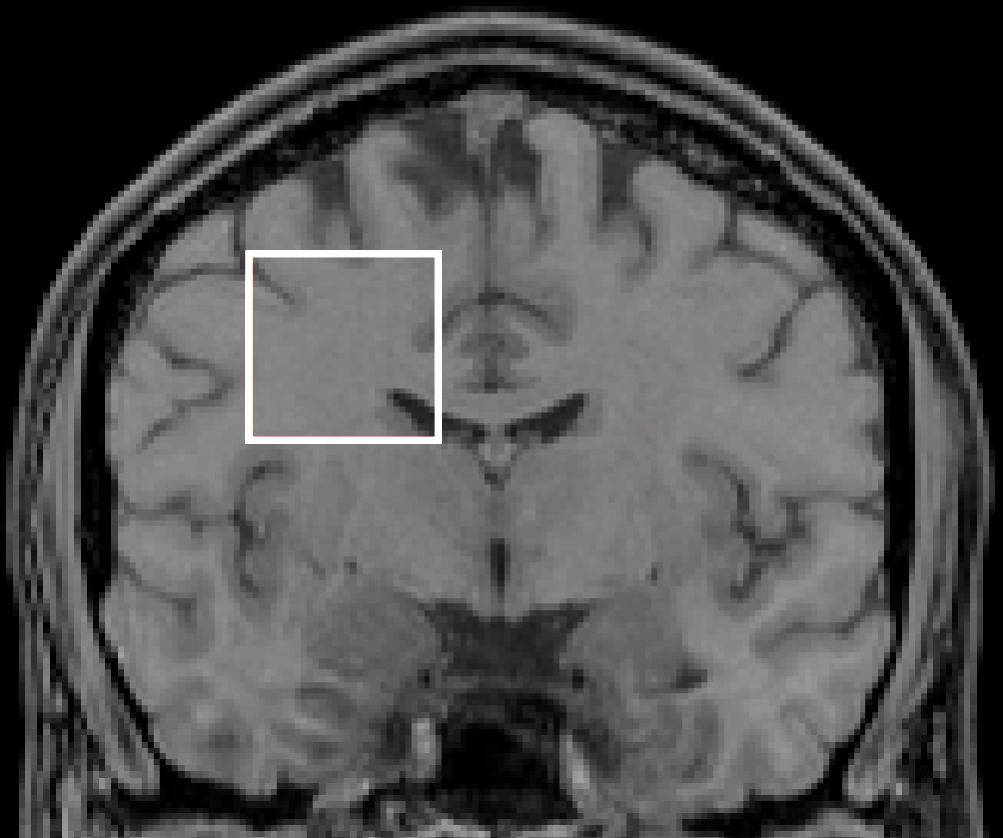where the blue curves are labeled as a crossing configuration, the green ones as a fan configuration, and the red ones as single, possibly curving, fibres. The image on the right shows the location of the ROI.

In , we show how the incorporation of this labeling information in a fibre tractography algorithm leads to improved results, as demonstrated in the following example: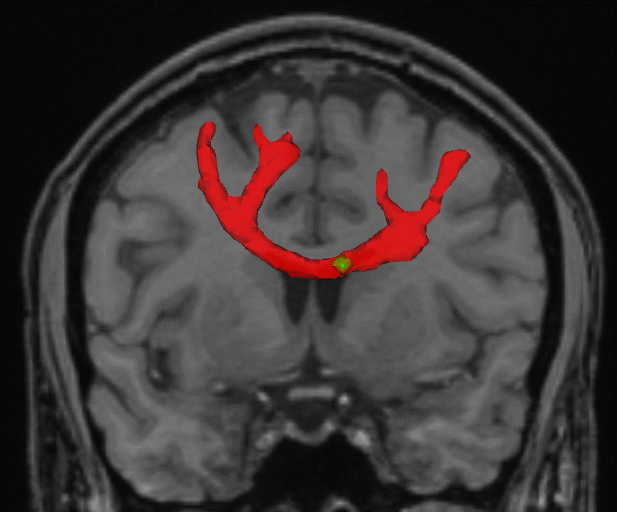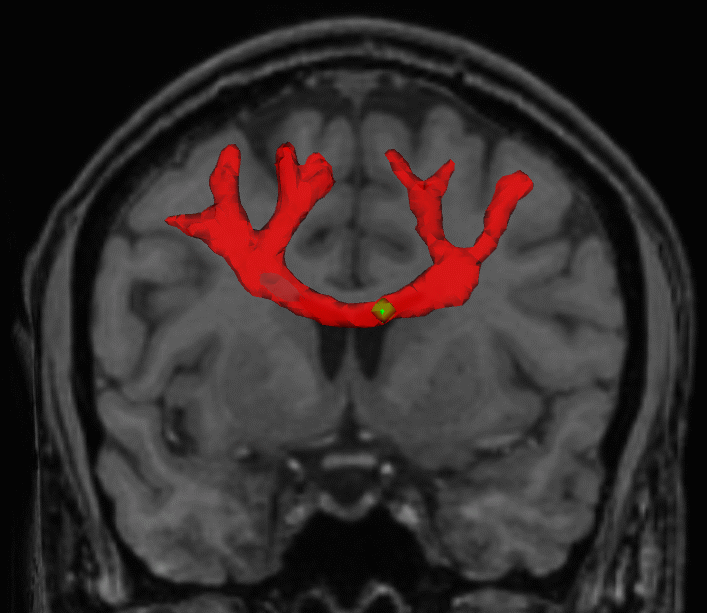Here, both images show the result of running a streamline tractography algorithm starting at the green seed located in the corpus callosum. The only difference is that the result on the left was obtained without the use of the labeling information. To obtain the result on the right, the tractography algorithm made use of the labeling information. One can see that the labeling allows tractography to follow fibre pathways closer to the cortex and to recover their fanning as they part to project to different neighbouring gyri. Please consult  for further details on this work.

### 3D streamline flows, generalized helicoids, and diffusion MRI analysis

3D streamline flows are sets of dense, locally parallel 3D curves. Such curve configurations arise in medical imaging, computer vision and graphics. The example that interests us most is that of white matter fiber tracts in diffusion MRI, but there are also texture patterns on surfaces, hair patterns in computer graphics, and 3D streamlines in visualization applications. Whereas the sense of a type of flow induced by such structures is immediate, as illustrated below, capturing the underlying geometry has proved difficult.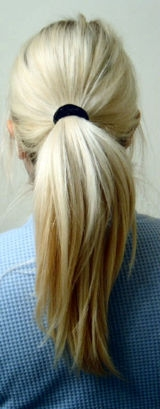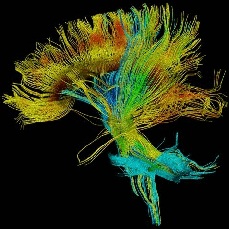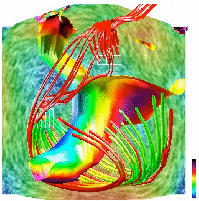The wide variety of methods proposed in the literature share the common theme of following only the streamline (tangent) direction. However, there is a rich geometric structure in the normal and bi-normal directions as well. For example, a fiber population in diffusion MRI, though approximately parallel locally, can diverge or branch and distinct fiber populations can cross. In work published in  and , we develop a differential geometric characterization of such structures by considering the local behaviour of the associated 3D frame field, which we then apply to the analysis of fibre tract geometry in diffusion MRI data.

A 3D streamline flow is represented by a frame field defined by the unit tangent, normal and bi-normal vectors (ET , EN, EB) at each point along each streamline. The use of a frame field allows for a natural representation of the geometric properties of the flow, since it is a generalization of the concept of the Frenet frame used to describe the geometry of individual curves.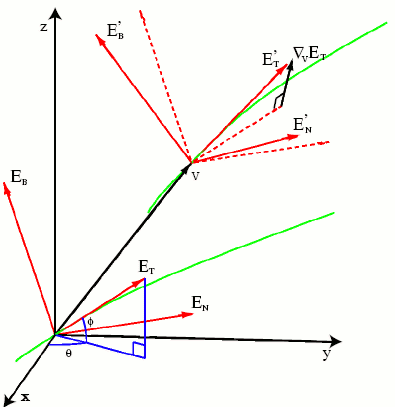In , we derive the three curvature functions that describe the behaviour of the (ET, EN, EB) frame as it moves within a small 3D neighborhood. Results from the theory of minimal surfaces motivate the choice of a combination of generalized helicoids as a "minimal" osculating object for 3D streamline flows, with their parameters related to the three curvature functions [6,7]. We observe that the curvature functions and the right helicoid osculating object presented in  for 2D texture flow are a special case of the equations derived in .

The inference of such an osculating object from in vivo diffusion MR data allows for the regularization and segmentation of white matter tracts, as demonstrated through a variety of experiments in [6,7]. Below, we present some examples.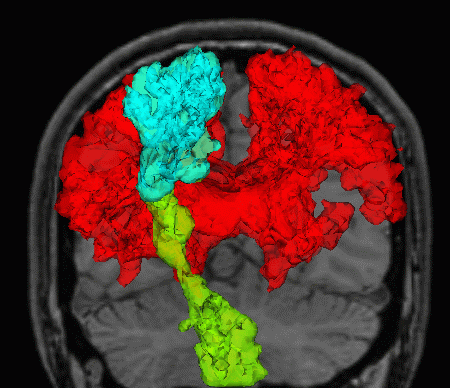The above figure is an example of white matter pathway segmentation obtained with an algorithm based on geometrical coherence with the inferred local streamline flow model , rather than on ODF shape statistics which may vary drastically along a given tract.

Below, we present regularization results obtained using 3D streamline flow inference. The fundamental idea is the same as that of 3D curve inference - at each voxel, support those orientations that are well supported in the neighborhood along the best-fit osculating object, and suppress the others.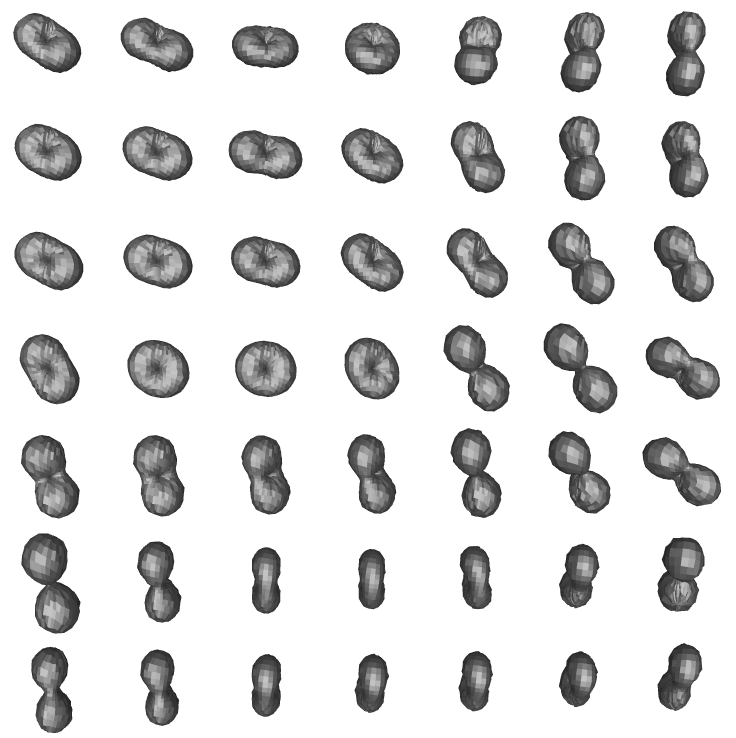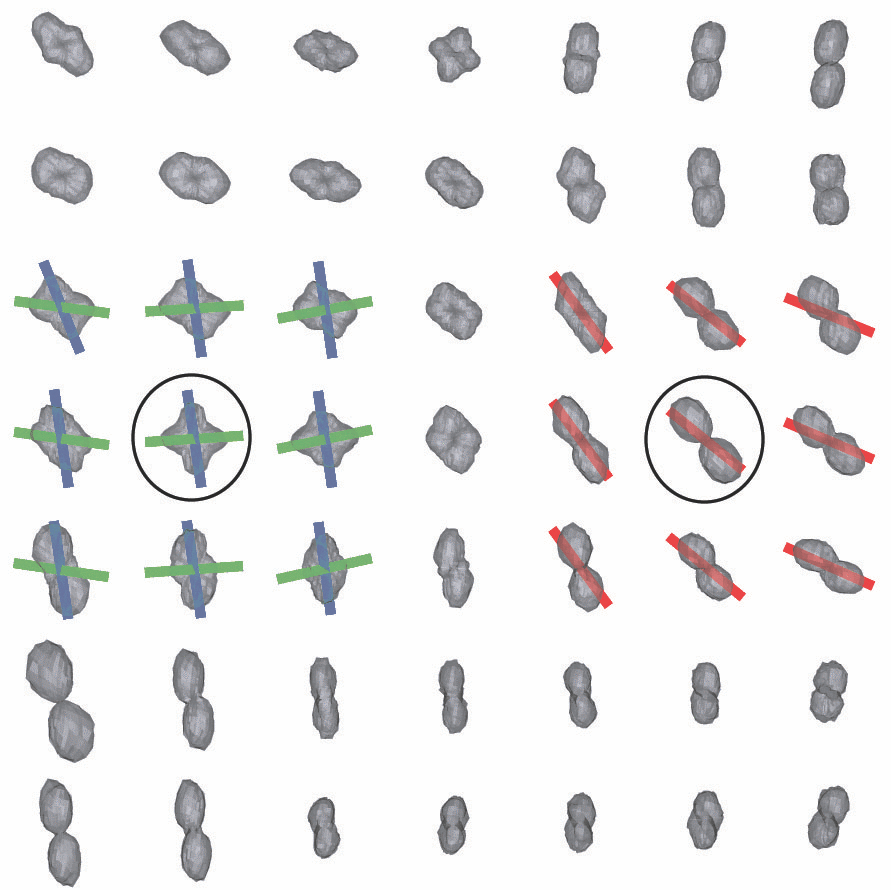The top row shows (from left to right): the original diffusion tensors (after subtraction of the maximally inscribed sphere for better visualization, see ), and the result obtained after applying the streamline flow based regularization. The bottom row shows (from left to right): the original QB ODF reconstruction, the result obtained after applying the same regularization algorithm, and the location of the ROI where all these results are observed. We observe that as expected, unregularized diffusion tensors are ambiguous in voxels where partial volume averaging of directions occurs. In contrast, both the QB and the regularized DT ODFs exhibit multiple maxima in these voxels that are qualitatively very similar to each other, just as was the case with 3D curve inference regularization. These figures also show the osculating objects associated with the ODF maxima in the circled voxels in red, green and blue. See  for further details.

### References

 P. Parent and S. W. Zucker. Trace inference, curvature consistency, and curve detection. IEEE Trans. Pattern Analysis and Machine Intelligence, 11:823-839, 1989.

 R. Hummel and S. W. Zucker. On the foundations of relaxation labeling processes. IEEE Trans. Pattern Analysis and Machine Intelligence, 5:267-287, 1983.

 O. Ben-Shahar and S.W. Zucker. The perceptual organization of texture flow: A contextual inference approach. IEEE Trans. Pattern Analysis and Machine Intelligence, 25:401-417, 2003.

 P. Savadjiev, J. S. W. Campbell, G. B. Pike, and K. Siddiqi. 3D curve inference for diffusion MRI regularization and fibre tractography. Medical Image Analysis, 10(5):799-813, October 2006. Download

 P. Savadjiev, J. S. W. Campbell, M. Descoteaux, R. Deriche, G. B. Pike, and K. Siddiqi. Labeling of ambiguous sub-voxel fibre bundle configurations in high angular resolution diffusion MRI. NeuroImage, 41(1):58-68, May 2008. Download

 P. Savadjiev, S. W. Zucker, and K. Siddiqi. On the differential geometry of 3D flow patterns: Generalized helicoids and diffusion MRI analysis. In IEEE Intl. Conf. on Computer Vision (ICCV)'07, 2007. Download

 P. Savadjiev, J. S. W. Campbell, G. B. Pike, and K. Siddiqi. Streamline flows for white matter fibre pathway segmentation in diffusion MRI. In MICCAI'08, 2008. Download